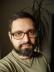## Perl Weekly Challenge 018/2: Priority Queue

Write a script to implement Priority Queue. It is like regular queue except each element has a priority associated with it. In a priority queue, an element with high priority is served before an element with low priority. Please check this wiki page for more information. It should serve the following operations:
1. `is_empty`: check whether the queue has no elements.
2. `insert_with_priority`: add an element to the queue with an associated priority.
3. `pull_highest_priority_element`: remove the element from the queue that has the highest priority, and return it. If two elements have the same priority, then return element added first.

## The Naive Implementation

If the priorities are non-negative integers and bounded by a reasonable maximum, the following implementation might be all you need. Let’s implement the queue as an array of arrays, each array element at position `\$p` represents all the queue elements with priority `\$p`.

Inserting into the queue is lightning fast, we just push to an inner array. Retrieving an element is slower, we have to find the first non-empty inner array and shift from it. To check whether the queue is empty we have to traverse all the inner arrays (but we could easily cache the number of elements the queue has).

``````package My::Queue::Priority::Array;

sub new { bless [], shift }

sub is_empty { ! grep @{ \$_ // [] }, @{ \$_ } }

sub insert_with_priority {
my (\$self, \$element, \$priority) = @_;
push @{ \$self->[\$priority] }, \$element;
}

sub pull_highest_priority_element {
my (\$self) = @_;
my (\$i) = grep @{ \$self->[\$_] || [] }, reverse 0 .. \$#\$self;
shift @{ \$self->[\$i] }
}``````

## Heap

The whole internet tells you that you should implement a priority queue with a heap. It works even for negative or floating point priorities.

I used a binary heap. It is a binary tree where each child has a lower priority than its parent. The element with the highest priority is stored in the root. To insert a new element, just add it as a child to an existing node that doesn’t have two children yet, and let it “bubble” up the tree, i.e. exchange it with its parent if the parent has lower priority, and repeat. Similarly, to extract the highest priority element, just remove the root of the tree. Move a leaf node into its position and let it “sink” into its position.

I implemented the binary heap in the traditional way: as an array where children of a node at position `\$p` are stored at positions `2 * \$p + 1` and `2 * \$p + 2`.

Unfortunately, it didn’t work exactly as requested: the last requirement from the specification wasn’t met; elements of the same priority were returned in random order. General heap isn’t stable.

To add stability, I introduced another another number stored with each element: the counter. It increases with each element stored to the heap, so if there are two elements of the same priority, we can compare their counters to see which one was introduced first. Note that the element with the higher priority gets pulled first, but for elements with the same priorities, we need to select the one with the lesser counter.

``````package My::Queue::Priority::Heap;
use enum qw( ELEMENT PRIORITY COUNTER );

sub new { bless [], shift }

sub is_empty { ! @{ \$_ } }

my \$i = 1;
sub insert_with_priority {
my (\$self, \$element, \$priority) = @_;
push @\$self, [\$element, \$priority, ++\$i];
my \$i = \$#\$self;
my \$p = int((\$i - 1) / 2);
while (\$p >= 0 && \$self->_compare(\$p, \$i)) {
@\$self[\$i, \$p] = @\$self[\$p, \$i];
\$i = \$p;
\$p = int((\$i - 1) / 2);
}
}

sub pull_highest_priority_element {
my (\$self) = @_;
my \$element = shift(@\$self)->[ELEMENT];
unshift @\$self, pop @\$self if @\$self;
return \$element
}

sub _compare {
my (\$self, \$p1, \$p2) = @_;
my \$c = \$self->[\$p1][PRIORITY] <=> \$self->[\$p2][PRIORITY];
return \$c == -1
|| \$c == 0 && \$self->[\$p1][COUNTER] > \$self->[\$p2][COUNTER]
}

my (\$self, \$pos) = @_;
if (my @children
= sort {
(\$self->[\$a][PRIORITY] <=> \$self->[\$b][PRIORITY])
|| (\$self->[\$b][COUNTER] <=> \$self->[\$a][COUNTER])
} grep defined \$self->[\$_],
2 * \$pos + 1, 2 * \$pos + 2
) {
if (\$self->_compare(\$pos, \$children[-1])) {
@\$self[\$pos, \$children[-1] ] = @\$self[\$children[-1], \$pos];
}
}
}``````

You can test your solution against the following test suite:

``````use Test::More;

my \$q = \$class->new();
ok \$q->is_empty;

\$q->insert_with_priority(@\$_)
for [a => 10], [b => 4], [c => 2], [d=>8], [e => 4], [f => 3];
ok ! \$q->is_empty;

for my \$e (qw( a d b )) {
is \$q->pull_highest_priority_element, \$e;
ok ! \$q->is_empty;
}

\$q->insert_with_priority(i => 1);
\$q->insert_with_priority(g => 4);
\$q->insert_with_priority(h => 5);

for my \$e (qw( h e g f c )) {
is \$q->pull_highest_priority_element, \$e;
ok ! \$q->is_empty;
}
is \$q->pull_highest_priority_element, 'i';
ok \$q->is_empty;

done_testing();``````I blog about Perl.